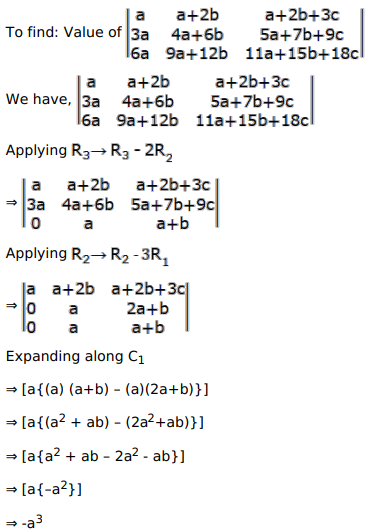# Mark the tick against the correct answer in the following:Question:

Mark the tick against the correct answer in the following:

$\left|\begin{array}{ccc}a & a+2 b & a+2 b+3 c \\ 3 a & 4 a+6 b & 5 a+7 b+9 c \\ 6 a & 9 a+12 b & 11 a+15 b+18 c\end{array}\right|=?$

A. $a^{3}$

B. $-a^{3}$

C. 0

D. none of these

Solution: